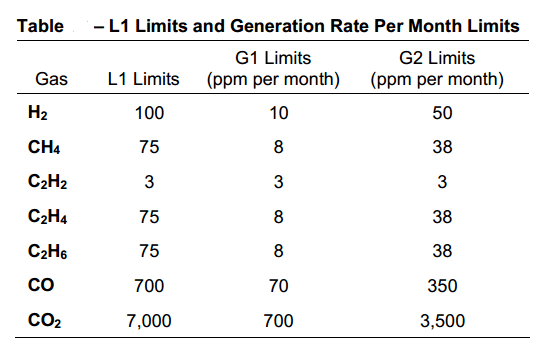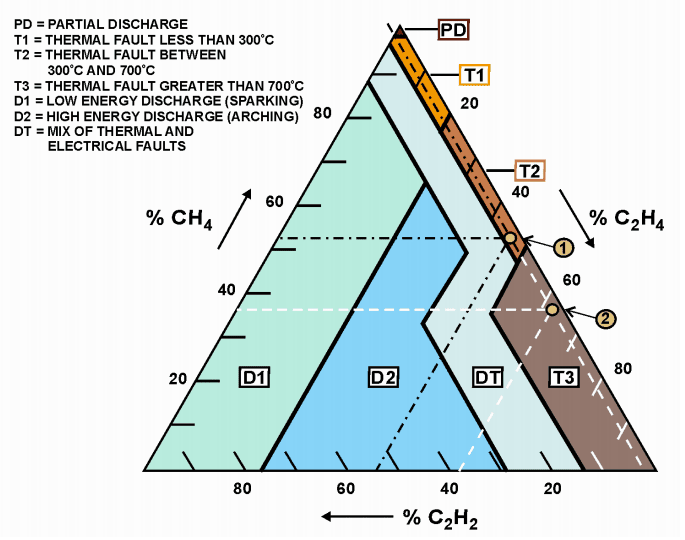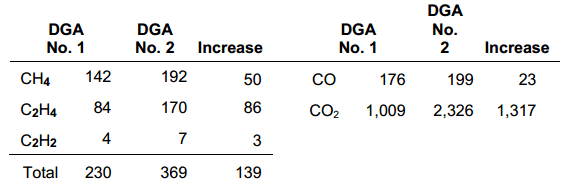# HOW TO DIAGNOSE TRANSFORMER ERRORS- USING DUVAL TRIANGLE

Table of content:

1. Origin of the Duval Triangle- diagnose transformer problems

2. How to use Duval's Triangle

3. Note

## 1. Origin of the Duval Triangle- diagnose transformer problems

Michel Duval of Hydro Quebec developed this method in the 1960s using a database of thousands of DGAs and diagnosing transformer failures or transformer problems. More recently, this method has been incorporated in Transformer Oil Analysis Software Version 4 (TOA 4), developed by Delta X Research and used by many in the utility industry to diagnose transformer failure. This method has proven to be accurate and reliable for many years and is now gaining popularity.The method and its use are described below.Picture 1: The Duval Triangle

## 2.How to use Duval's Triangle

2.1 First, determine transformer problem using the IEEE® method above and/or the table shown in Figure 2 below. At least one of the hydrocarbons or hydrogen must be in condition IEEE® 3 and increasing at the rate of generation (G2), from the table shown in Figure 2, before the problem is confirmed. To use this table without the IEEE® method, at least one of the individual gases must be L1 or higher and the gas generation rate must be at least G2. The L1 limits and gas generation rates from the table in Figure 2 are more reliable than those of the IEEE® method; however, one should use both methods to confirm that a problem exists. Suppose there is a sudden increase in H2 with only carbon monoxide and carbon dioxide and little or no hydrocarbon gas. In that case, we need to determine if the cellulose insulation has decomposed by overheating.

2.2 Once the transformer problem has been determined to exist, use the cumulative sum of the three Duval Triangle gases and draw the percentage diagram of the total over the triangle to arrive at a diagnosis. An example is shown below. Also, calculate the amount of the three gases used in the Duval Triangle, produced since the start of the sudden increase in the amount of gas. Subtracting the amount of gas produced before the sudden increase will give the amount of gas produced since the incident started. Detailed instructions and an example are shown below.

a. Take the amount (ppm) of methane in the DGA and subtract the amount of CH4 in the previous DGA, before the gas spiked. This will give the amount of methane produced since the incident started.

b. Repeat this process for the remaining two gases, ethylene and acetylene.

2.3 Add the three numbers (differences) obtained using the procedure in step 2 above. This produces 100% of the three main gases, generated from breakdown, used in the Duval Triangle.

2.4 Divide the difference of each individual gas by the total difference of gases obtained in step 3 above. This shows the percentage increase of each gas in the total increase.

2.5 Plot the percentiles of each gas on the Duval Triangle, starting at the side indicated for that particular gas. Draw lines across the triangle for each gas, parallel to the sharp signs shown on each side of the triangle. An example is shown below.Figure 2: L1 Limit and Monthly Generation Rate Limit

CAUTION: Do not use the Duval Triangle (Figure 1) to determine if a transformer has a fault. Note that there is no area on the triangle for a fault-free transformer. The triangle will show the error for every transformer whether it has a fault or not. Use the IEEE method above or the table in Figure 2 to determine if a problem exists before applying Duval Tirangle. The Duval Triangle is only used to determine what the problem is. As with the other methods, a significant amount of gas must be present (at least the L1 limit and G2 generation rate in the table in Figure 2) before this method becomes effective.

## 3. NOTE:

In most cases acetylene will be zero and the result will be a point on the right side of the Duval Triangle.

Compare the cumulative total gas diagnosis and the diagnosis obtained using only the post-fault gas rise. If the error has existed for a while or if the incidence is high, then the two diagnoses will be the same. If the diagnoses are not the same, always use the diagnosis of an increase in the amount of gas produced by the error, which will be the more severe of the two. See an example of a transformer below where the diagnostic uses more severe gas increases than when the total accumulated gas is used.Figure 3: Example of diagnosis of Duval's triangle

Example:

Using Figure 3 and the information below (Figure 4), two transformer diagnostics were obtained. The first diagnosis (Score 1) is obtained using the sum of the three gases used by the Duval Triangle. The second diagnosis (Score 2) was obtained only using the gas rise between the two DGAs. CO and CO2 were used to evaluate cellulose.Image 4: DGA . board

Steps to get the first diagnosis (Point 1) on the Duval Triangle (Figure 3):

1. Using total gas accumulated from DGA 2 = 369

2. Divide each gas by the sum to find the percent composition of each gas in the total.

%CH4 = 192/369 = 52%, %C2H4 = 170/369 = 46%,

%C2H2 = 7/369 = 2%

3. Draw three lines across the Duval Triangle starting from the percentage obtained in step 2. These lines should be drawn parallel to the hash mark on each respective side. See the black dashed lines in Figure 1 above.

4. Score 1 is obtained when the lines intersect in T2 diagnostic region of the triangle, which indicates a thermal error of 300 to 700 °C. See Figure 1, Legend, above.

Steps to obtain a second diagnosis (Point 2) on the Duval Triangle (Figure 3):

1. Use total gas increase = 139.

2. Divide each increment by the total increment to find the % composition of each gas in the total:

% increase CH4 = 50/139 = 36%

% increase C2H4 = 86/139 = 46%

% increase C2H2 = 3/139 = 2%

3. Draw three lines across the Duval Triangle starting from the percentage obtained in step 2. These lines should be drawn parallel to the pound sign on each respective side. See the white dashed lines in Figure 3 above. Note that C2H2 has the same percentage (2%) both times, and thus both lines are the same.

4. Score 2 is obtained when the lines intersect in the T3 diagnostic region of the triangle, which indicates a thermal error greater than 700°C. See Figure 1, Caption, above.

The ratio of total gas accumulation is CO2 / CO = 2.326 / 199 = 11.7. The rate of increase is CO2/CO = 1.317/23 = 57. Neither of these ratios is low enough to be cause for concern. This indicates that the thermal fault is not close enough to the cellulose insulation to cause thermal deterioration of the insulation. A large increase in CO2 can mean a leaky atmosphere.

3.NOTE:

1. Score 2 is a more severe diagnosis obtained using increased gas volume rather than total gas accumulation. It would be helpful to do both methods as a check. Many times both diagnoses will be the same.

2. CO and CO2 included show that the fault is not related to severe degradation of the cellulose insulation.

The failure is base on bad connection at the bottom of the bushing, a bad contact or connection in the faucet changer or a problem with the core ground. These problems are probably all fixable in this regard. Any of these problems can cause the results revealed by the Duval Triangle diagnosis above. These are areas where failure will not degrade the cellulose insulation, which will result in a much lower CO2/CO ratio than what is obtained.

Related article:

three phase transformers

transformer rating

losses in transformer

potential transformer

rmu

rmu electrical

Required professional transformer knowledge

A transformer expert should be consulted if some DGA justifies the problem. The transformer manufacturer should be consulted with DGA laboratory personnel and others experienced in transformer maintenance and diagnostics. Never diagnose based on a DGA. A sample may have been mishandled or mislabeled, either in the field or in the laboratory.

.(+84) 0913.006.538
Skype, Telegram, Wechat ...